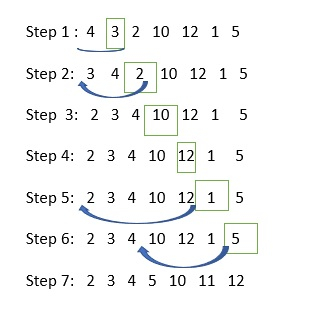# Explain the insertion sort by using C language.

Sorting is the process of arranging the elements either in ascending (or) descending order.

## Types of sorting

C language provides five sorting techniques, which are as follows −

• Bubble sort (or) Exchange Sort
• Selection sort
• Insertion sort(or) Linear sort
• Quick sort (or) Partition exchange sort
• Merge Sort (or) External sort

## Insertion Sort

The logic used to sort the elements by using the insertion sort technique is as follows −

for(i = 1; i <= n - 1; i++){
for(j = i; j > 0 && a[j - 1] > a[j]; j--){
t = a[j];
a[j] = a[j - 1];
a[j - 1] = t;
}
}

## Explanation

Let us consider some elements which are in unsorted order −## Example

Following is the C program to sort the elements by using the insertion sort technique −

#include<stdio.h>
int main() {
int a, i,j,n,t;
printf("enter the No: of elements in the list:");
scanf("%d", &n);
printf("enter the elements:");
for(i=0; i<n; i++){
scanf ("%d", &a[i]);
}
for(i = 1; i <= n - 1; i++){
for(j=i; j > 0 && a[j - 1] > a[j]; j--){
t = a[j];
a[j] = a[j - 1];
a[j - 1] = t;
}
}
printf ("after insertion sorting the elements are:");
for (i=0; i<n; i++)
printf("%d\t", a[i]);
return 0;
}

## Output

When the above program is executed, it produces the following output −

Enter the No: of elements in the list:
10
Enter the elements:
34
125
2
6
78
49
1
3
89
23
After insertion sorting the elements are:
1 2 3 6 23 34 49 78 89 125